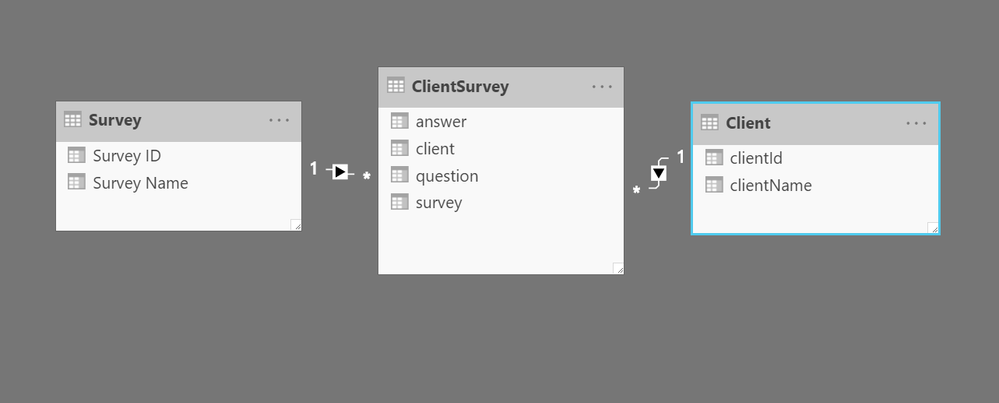cancel
Showing results for
Did you mean:Helper I

## DAX help

Hi All,

I am new to DAX and I have a table with losts of different surveys and peoples answers to the surveys.

1) I need to know if a customer has completed all of the questions (in this example 8 questions)

2) If all questions are answered mark the survey as complete.

3) count that as one completed survey.

4) If the survey has one question answered mark as part completed, and count as one servey (to count the servey I would just count (distinct) customerID)Could anyone give me some guidance on how I would acheive this please?

Many thanks,

Sean.

1 ACCEPTED SOLUTIONSolution Sage

The DAX will be much easier if you break your one big table down into a star schema (I can help you break it down if you need)My next step was to add a calculated column to the Client Survey records to determine if an individual question had been answered.
Here's the DAX for that column:

Once I have that I can create a visualThe visual uses 2 measures, one that counts the number of questions for each survey, the other that counts the number of answers:
Number Questions = countrows(ClientSurvey)
Then I can subtract them to see if the survey is complete
survey complete = AND([Number Questions]>0,[Number Questions] = [Number Answers])
or to see if it was partial
survey partial = AND([Number Questions]>0, [Number Answers]>0)

that's a lot all at once. i'd be glad to do a screen share and walk through it with you. send me your email and i'll send you a zoom invitation. I'll be free in about an hour and a half

I'm a personal Power BI trainer  every time I answer a question I learn something

Did this post answer your question? Mark it as a solution so others can find it!

Help when you know. Ask when you don't!

Join the conversation at We Talk BI find out more about me at Slow BI

2 REPLIES 2Solution Sage

The DAX will be much easier if you break your one big table down into a star schema (I can help you break it down if you need)My next step was to add a calculated column to the Client Survey records to determine if an individual question had been answered.
Here's the DAX for that column:

Once I have that I can create a visualThe visual uses 2 measures, one that counts the number of questions for each survey, the other that counts the number of answers:
Number Questions = countrows(ClientSurvey)
Then I can subtract them to see if the survey is complete
survey complete = AND([Number Questions]>0,[Number Questions] = [Number Answers])
or to see if it was partial
survey partial = AND([Number Questions]>0, [Number Answers]>0)

that's a lot all at once. i'd be glad to do a screen share and walk through it with you. send me your email and i'll send you a zoom invitation. I'll be free in about an hour and a half

I'm a personal Power BI trainer  every time I answer a question I learn something

Did this post answer your question? Mark it as a solution so others can find it!

Help when you know. Ask when you don't!

Join the conversation at We Talk BI find out more about me at Slow BIHelper I

Thank you for the asnwer, I will try and understand it in the morning.